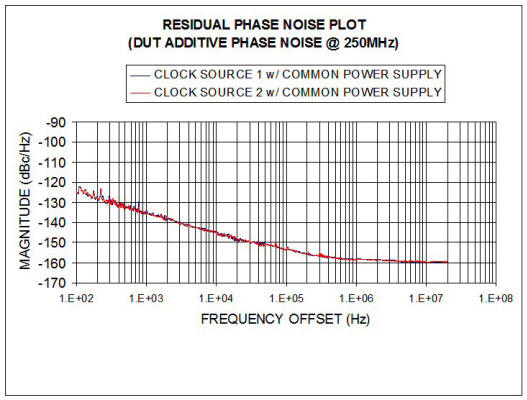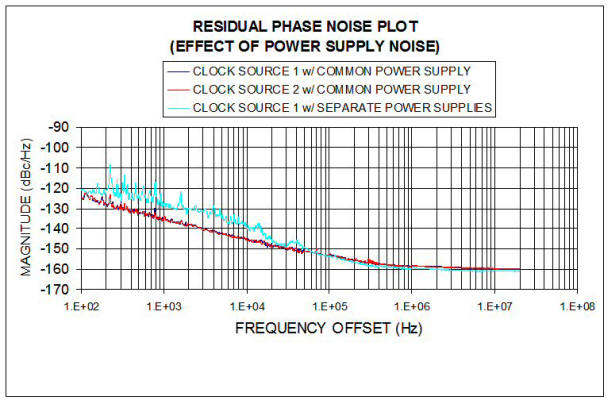# Residual Phase Noise Measurement Extracts DUT Noise from External Noise Sources

Residual phase noise measurement cancels the effect of external noise sources, such as power supplies or input clocks, as opposed to absolute phase noise measurement, which includes the noise from these sources. A residual phase noise setup isolates and measures a device’s additive phase noise. Using this information, designers select individual devices in the signal chain in order to meet the phase noise requirements of the complete system. This article includes phase noise plots of a clocked device to highlight the attributes of the residual phase noise setup. It also demonstrates how a device’s additive phase noise can be used to identify the source of noise-related issues in the signal chain.

Figure 1 shows the setup used to measure the additive phase noise of the device under test (DUT). Note that two DUTs are used; each is connected to a common power supply and input clock. The phase noise due to these common noise sources is correlated at each DUT output. The output phase noise can be derived by simply modeling the phase detector as an analog multiplier with gain KPD:

where E1 is the amplified DUT1 output signal, E2 is the amplified and delayed DUT2 output signal, EC1 and EC2 are the signal powers, θM1 and θM2 are the magnitudes of the phase noise, ωC is the carrier frequency, and ωM is the offset frequency. Superposition applies, so the phase noise intrinsic to the DUTs can be neglected when considering the phase noise from external sources. If DUT1 and DUT2 have identical excess phase transfer functions, the portion of θM1 due to the clock source and power supplies is equal to the portion of θM2 due to the common clock source and power supplies. This phenomenon, supply pushing, is simply described by:

such that the magnitude of the phase modulation is given by the product of the voltage noise on the supply and KP, the pushing gain in radians/V. If DUT1 and DUT2 have equivalent pushing gains, these noise sources are theoretically cancelled at the phase detector’s output, leaving only the uncorrelated noise of the two DUTs for measurement.

The intrinsic DUT noise can be ascertained with a few additional assumptions. Since the rms phase error due to device noise is generally very small, we can rewrite the expression for the output carrier using the small-angle approximation as:

The output of the phase detector has been demodulated, so it can be called the baseband signal. The actual phase noise can be calculated once the phase detector gain and the input signal power have been determined, assuming that the amplifier phase noise contribution is negligible. The noise intrinsic to each DUT is uncorrelated, so they contribute equally, and the rms sum is the measured output phase noise. For this reason, we subtract 3 dB from the phase noise measured by the spectrum analyzer (in dBc/Hz) to determine the contribution from each DUT. This represents the phase noise power relative to the signal power:

When making very sensitive phase noise measurements, the noise contribution of the amplifier may be significant. The residual phase noise of the amplifiers is measured by removing DUT1 and DUT2 from the circuit and applying the power splitter outputs directly to the amplifiers. The amplifier input signal power must resemble the actual DUT output signal in amplitude and slew rate. Using the procedure described above, the measured amplifier phase noise is subtracted from the measured DUT phase noise to obtain the precise DUT phase noise. Again, it is important that the gain and noise figure of the amplifiers resemble one another as closely as possible.

Note that a DUT that requires clocking will have a front end amplifier that exhibits a certain amount of noise. For this reason, a clock source with a low slew rate could unintentionally increase the DUT’s phase noise contribution due to threshold uncertainty at the amplifier input. When using a sinusoidal clock source, maximize the slew rate by using the maximum allowable amplitude.

### Basic details of the test setup

Using the test setup from figure 1, two DUTs with the same part number were clocked by a single 1-GHz source. The devices were set to divide the clock frequency by four to produce a 250-MHz output. In addition, the two output signals were shifted in relative phase by 90° (quadrature) to minimize the down-converted signal level appearing at dc. The DUT signals were amplified by a low noise amplifier (LNA) to increase the dynamic range of the measurement system (the phase noise contributed by the amplifiers is small enough to be ignored). The amplifier outputs were sent to a balanced mixer (phase detector). The phase detector mixes the two signals, producing sum and difference products at its output. The sum product was filtered out with a low-pass filter. The remaining difference product constitutes the 250-MHz output signal down-converted to dc (phase noise). The LNA provided sufficient gain to overcome the noise floor limitation of the spectrum analyzer.

### Cancellation of common clock source phase noise

Figure 2 shows the absolute phase noise measurement of two clock sources that exhibit very different phase noise characteristics. Theoretically, neither clock source should impact the DUTs additive phase noise measured using a residual phase noise setup. Figure 3 confirms this theory. Two separate residual phase noise measurements are plotted, one trace for each clock source. The two traces virtually overlap, proving that the common clock source noise is cancelled by the residual phase noise setup. This noise would not be cancelled in an absolute phase noise setup. In fact, if the DUTs were ideal (no additive phase noise), their absolute phase noise curves would match the curves in Figure 2 (but 12 dB lower due to the factor-of-four frequency translation). Normalized to a 250-MHz carrier, clock source 2 exhibits –92 dBc/Hz phase noise at 1 kHz offset, whereas the measured DUT phase noise associated with clock source 2 is –135 dBc/Hz at 1 kHz. The residual phase measurement thus suppressed approximately 40 dB of the input clock phase noise.Figure 2. Absolute phase noise measurement of two different clock sources.Figure 3. Clock source has virtually no impact on the residual phase noise measurement.

### Cancellation of common power supply noise

Figure 3 uses a common power supply connection as shown in Figure 1. Figure 4 shows the effect of using separate noisy power supplies for each DUT. The uncorrelated power supply noise causes a substantial increase in the close-in phase noise.Figure 4. Residual phase noise measurement displays the impact from common and separate power supplies.

Figure 5 shows the absolute phase noise measurement using a low-noise power supply. The absolute phase noise with low-noise power supply and the residual phase noise with separate low-noise power supplies show good agreement. The power supply phase noise is cancelled in the residual phase noise measurement but not in the absolute phase noise measurement.Figure 5. Close-in phase noise improvement due to low-noise power supply

The residual phase noise measurement is a valuable technique used to identify the phase noise contribution of a single component as part of a system design. Using this approach, external noise sources, such as the input clock and power supply, are correlated at each DUT output, and can therefore be effectively cancelled. Furthermore, it is possible to account for the phase noise contribution of buffers or amplifiers used in the DUT residual noise measurement by performing additional residual phase noise measurements on these components. Combining residual and absolute phase noise measurements is a powerful way to identify the dominant noise source in a system design. The presented measurement data, acquired on a frequency divider, demonstrates the concept and utility of the residual phase noise measurement, with the effects of a noisy input clock and power supply have been quantified. From this evaluation, a system designer can derive specifications for the input clock source and power supplies based on actual measurement data.